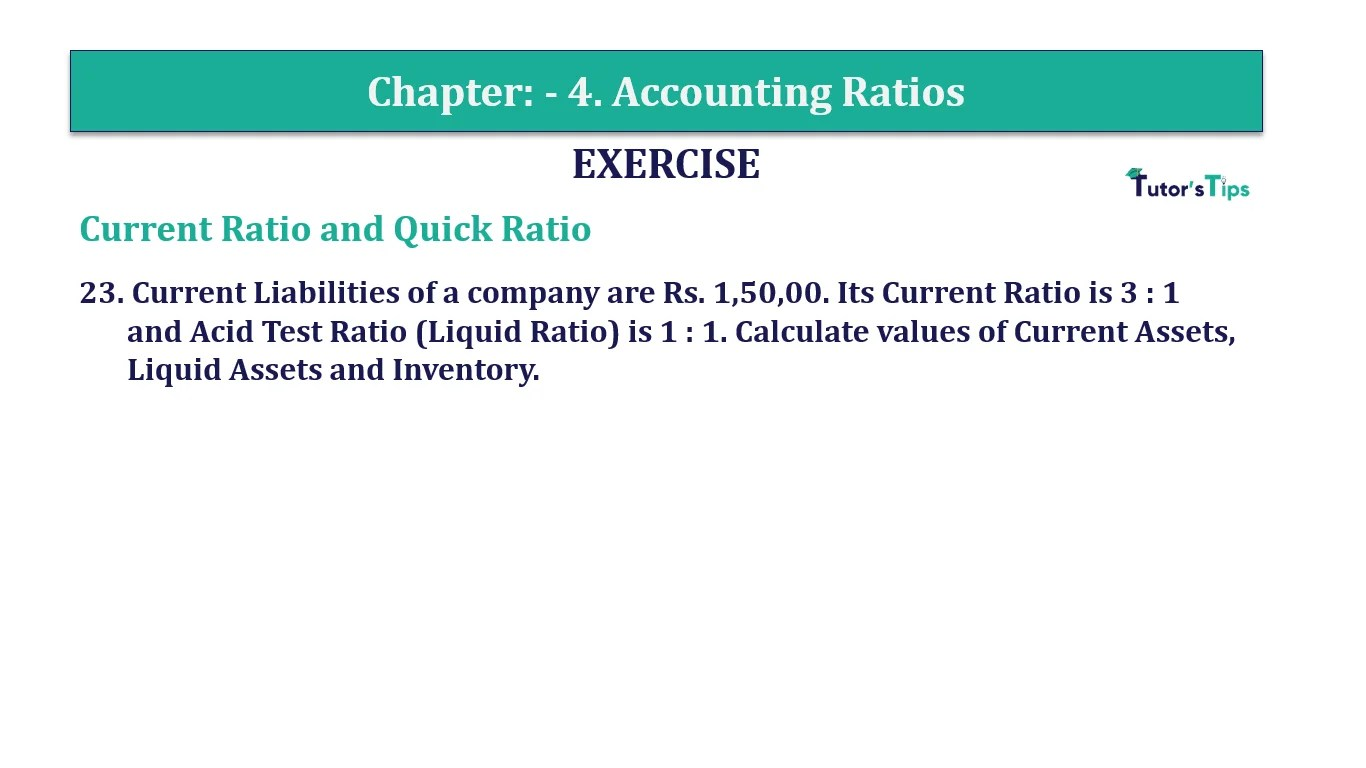# Question 23 Chapter 4 of +2-B – T.S. Grewal 12 ClassQuestion No. 23 - Chapter No.4 - T.S. Grewal +2 Book Part B

Question 23 Chapter 4 of +2-B

Current Ratio and Quick Ratio

23. Current Liabilities of a company are Rs. 1,50,00. Its Current Ratio is 3 : 1
and Acid Test Ratio (Liquid Ratio) is 1: 1. Calculate values of Current Assets, Liquid Assets and Inventory.

### The solution of Question 23 Chapter 4 of +2-B: –

 Current Ratio = Current Assets = 3 Current Liabilities 1

 Liquid Ratio = Liquid Assets = 1 Current Liabilities 1
 Current Liabilities = Rs. 1,50,000 Current Assets = 3 x Current Liabilities 3 x 1,50,000 = Rs. 4,50,000 Liquid Assets = 1 x Rs.1,50,000 = Rs. 1,50,00 Inventories = Current Assets – Liquid Assets = Rs. 4,50,000 – 1,50,000 = Rs. 3,00,000

Balance Sheet: Meaning, Format & Examples

Comment if you have any question.

Also, Check out the solved question of previous Chapters: –